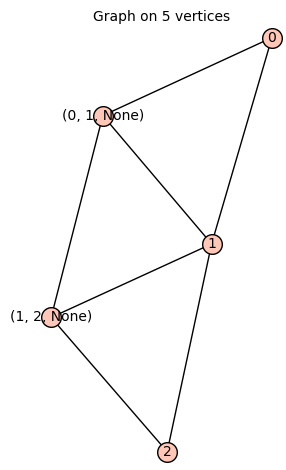# Constructing total graph

Let $G$ be a graph of order $n$ and size $e$. The total graph $T$ is the graph associated to $G$, which consists of order $n+e$ and edges consist of the union of the edges of $G$, the edges of line graph of $G$ and the edges of the subdivision graph of $G$.

I know that there is the line_graph() module in SageMath. But, I am unaware of the any module for subdivision graph. In addition, how do we form a disjoint union of edges of the three graphs $G$, its line and subdivision graphs, when their vertex sets are different. So finally, how can we construct the total graph $T$ in SageMath. Any hints? Thanks beforehand.

edit retag close merge delete

Sort by » oldest newest most votedThere is a subdivide_edges method but it doesn't seem to give control over the names of the new vertices.

If I understood the definition correctly then the implementation can go like this:

def total_graph(G):
T = G.union(G.line_graph())
for (a,b,l) in G.edges():
return T


Example 1:

sage: graphs.PathGraph(2).edges()
[(0, 1, None)]
sage: total_graph(graphs.PathGraph(2)).edges(sort=False)
[(0, 1, None), ((0, 1, None), 0, None), ((0, 1, None), 1, None)]


Example 2:

sage: graphs.PathGraph(3).edges()
[(0, 1, None), (1, 2, None)]
sage: total_graph(graphs.PathGraph(3)).edges(sort=False)
[((0, 1, None), (1, 2, None), None), (0, 1, None), ((0, 1, None), 0, None), (1, 2, None), ((0, 1, None), 1, None), ((1, 2, None), 1, None), ((1, 2, None), 2, None)]
sage: total_graph(graphs.PathGraph(3))more

Thanks! This uses looping unlike my answer, which uses the subdivide_edges method

By using the equivalent definition of total graph as the square(or distance-2 graph) of the subdivision graph(the graph formed by subdividing each edge, we obtain the following pseudo-code:

def TotalGraph(G):
h=G
k = 1
G.subdivide_edges(G.edges(), k)
h=G.distance_graph(list(range(1,3)))
return h


This seems to work in my case.

more